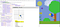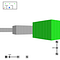# Composability and the builder monad

`reflexOf :: (_ => ReactiveInput t -> m (Dynamic t Picture)) -> IO ()`
`class ReflexCodeWorld t mgetKeyPress :: ReflexCodeWorld t m => m (Event t Text)getKeyRelease :: ReflexCodeWorld t m => m (Event t Text)getTextEntry :: ReflexCodeWorld t m => m (Event t Text)getPointerClick :: ReflexCodeWorld t m => m (Event t Point)getPointerPosition :: ReflexCodeWorld t m => m (Dynamic t Point)isPointerDown :: ReflexCodeWorld t m => m (Dynamic t Bool)getTimePassing :: ReflexCodeWorld t m => m (Event t Double)draw :: ReflexCodeWorld t m => Dynamic t Picture -> m ()reactiveOf :: (forall t m. ReflexCodeWorld t m => m ()) -> IO ()`
`{-# LANGUAGE OverloadedStrings #-}import CodeWorld.Refleximport Data.Text (Text)import Reflexmain :: IO ()main = reactiveOf \$ do    up <- button "Up" (0, -4)    down <- button "Down" (0, -8)    left <- button "Left" (-4, -6)    right <- button "Right" (4, -6)    pos <- foldDyn (\$) (0, 0) \$ mergeWith (.) [        (\(x, y) -> (x, y + 1)) <\$ up,        (\(x, y) -> (x, y - 1)) <\$ down,        (\(x, y) -> (x + 1, y)) <\$ right,        (\(x, y) -> (x - 1, y)) <\$ left        ]    draw \$ uncurry translated <\$> pos <*> pure (solidCircle 0.2)    return ()button :: ReflexCodeWorld t m => Text -> Point -> m (Event t ())button label (bx, by) = do    draw \$ constDyn \$ translated bx by \$        dilated 0.75 (lettering label) <>        rectangle 3 1 <>        colored (light gray) (solidRectangle 3 1)    click <- getPointerClick    return (() <\$ ffilter onButton click)  where onButton (x, y) = abs (x - bx) < 1.5 && abs (y - by) < 0.5`
`button :: ReflexCodeWorld t m => Text -> Point -> m (Event t ())button label (bx, by) = do    hover <- fmap onButton <\$> getPointerPosition    let color = bool (light gray) (light (light gray)) <\$> hover    draw \$ pic <\$> color    click <- getPointerClick    return (() <\$ ffilter onButton click)  where    onButton (x, y) = abs (x - bx) < 1.5 && abs (y - by) < 0.5    pic color = translated bx by \$        dilated 0.75 (lettering label) <>        rectangle 3 1 <>        colored color (solidRectangle 3 1)`

# More Effects

`{-# LANGUAGE JavaScriptFFI #-}import CodeWorld.Refleximport Control.Monad.Trans (liftIO)import Reflexforeign import javascript unsafe "alert(\$1 + ',' + \$2)"    alert :: Double -> Double -> IO ()main :: IO ()main = reactiveOf \$ do    click <- getPointerClick    performEvent_ \$ (\(x, y) -> liftIO \$ alert x y) <\$> click`

# FRP and DebuggingThe CodeWorld InspectorOn-screen debug controls in a CodeWorld program

# What else?

Written by

## Chris Smith

#### Software engineer at Google, volunteer math and computer science teacher, author of the CodeWorld platform, amateur ring theorist, and Haskell enthusiast.

Welcome to a place where words matter. On Medium, smart voices and original ideas take center stage - with no ads in sight. Watch
Follow all the topics you care about, and we’ll deliver the best stories for you to your homepage and inbox. Explore
Get unlimited access to the best stories on Medium — and support writers while you’re at it. Just \$5/month. Upgrade